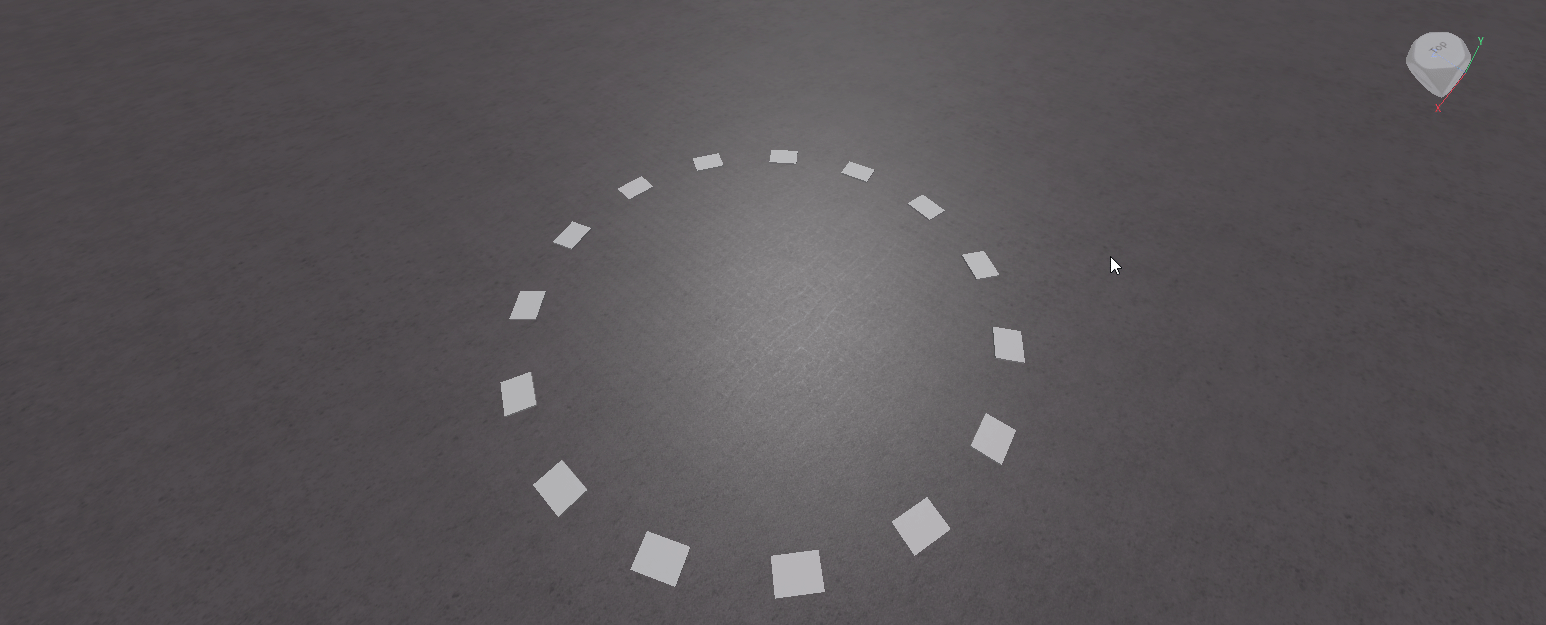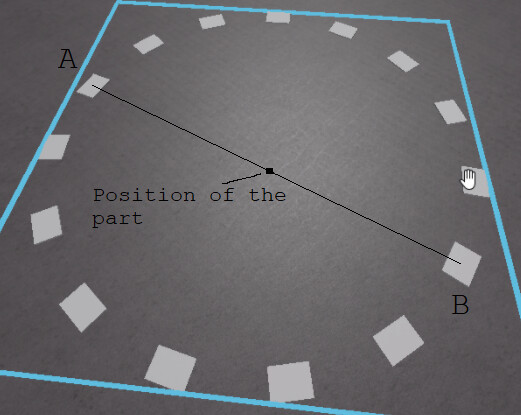# Is there way to rotate model with parts?

Hello!
I’m currently wondering how to make rotate parts in model like thisI tried used :SetPrimaryPartCFrame() but that did now work well
Thanks for any help!

1 Like

Having all the parts modeled together is a nice workaround. Just include a part that’s exactly in the middle which will be the primary part, and hopefully you guessed it, you’ll be `model:SetPrimaryPartCFrame()`ing it with a rotation CFrame each time.

The easiest way to do insert this part perfectly in the center of the cricle, is to position it in the middle of two opposite parts. The two opposite parts represent a diameter in the circle, and the middle of a diameter is the center of that circle. While in edit mode, you rename two of parts that are opposite of each other temporarly so we know which ones we’re working with, inserting a third part (using the command bar of course! This is all done in edit mode) in the middle is as simple as (`A` and `B` are two opposite parts we picked):

``````Instance.new("Part", workspace).Position = workspace.A.Position:Lerp(workspace.B.Position, 0.5)
``````

We create a new part parented to workspace (`Instance.new("Part", workspace)`) and directly position it to the middle, which is (`workspace.A.Position:Lerp(workspace.B.Position, 0.5)`). Lerping can be thought of as the percentage of how far we want to go from A to B, we want to go half way (`0.5`) from A to B, and it’s quite clear that’s the middle of them. Feel free to ask if you didn’t understand.After that you may want to do some stuff to the created part, set it as a primary part first, anchor it, uncancollide it, make it transparent ect. (Of course don’t change the position)

Rotating now is just

``````while true do
wait()
end
``````

This is just for demonstration! You’re not gonna want to use a while loop with a wait() in your final product.

Each time, we set the primary part cframe to the previous cframe, rotated by (which is multiplying by a `CFrame.Angles`) `0.1` degrees along the X axis (if it doesn’t work rotate the Z axis instead, I’m a dummy!)

A much nicer solution, that doesn’t use a model, will just be simple trigonometry, converting polar coordinates to cartesian ones, but explaining this is hmm, yeah. If you’re interested, I’m happy to explain.
Have all the parts under a certain folder, or just have references to them in a table.

``````local parts = workspace.Folder:GetChildren() --this are our parts

local radius = 10 --radius of the circle, not sure what you chose in the pic!
local angle = 0
for i, part in parts do
part.Position = Vector3.new(r * math.cos(angle) , 1, r * math.sin(angle)) --1 is an arbitrary value for the Y axis
end``````
1 Like

The best way to do is use Colbert2677’s method of tweening models. Put an invisible part in the middle of your circle(model), that part will be the pivot(must be anchored!)
now add a weld constraint to each of the circle parts except the pivot and weld to the pivot. btw your parts that are welded to the pivot should be unanchored so when the pivot rotates, the parts will also follow along because its welded
now use Tween service to rotate the pivot

``````local pivot = pathtoyourpivot
local tweenservice = game:GetService("TweenService")
local tweenanimation = TweenInfo.new(2,Enum.Easingstyle.Linear)-- any choice

while true do
local goal = {CFrame = pivot.CFrame*CFrame.Angles(0,math.rad(-180),0)} -- use 180 degress otherwise 360* wont rotate because its the same as rotating 0 degrees
local tween = tweenservice:Create(pivot,tweenanimation,goal)
tween:Play()
tween.Completed:Wait()
end
``````

``````local parts = YourModel:GetChildren()